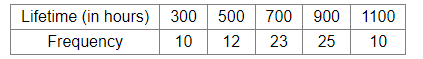# 80 bulbs are selected at random from a lot and their lifetime in hours is recorded as under.Question:

80 bulbs are selected at random from a lot and their lifetime in hours is recorded as under.One bulb is selected at random from the lot. What is the probability that the selected bulb has a life more than 500 hours?

(a) $\frac{27}{40}$

(b) $\frac{29}{40}$

(c) $\frac{5}{16}$

(d) $\frac{11}{40}$

Solution:

(b) $\frac{29}{40}$

Explanation:
Total number of bulbs in the lot  = 80
Number of bulbs with life time of more than 500 hours = (23 + 25 + 10) = 58

Let E be the event that the chosen bulb's life time is more than 500 hours.

$\therefore$ Required probability $=P(E)=\frac{58}{80}=\frac{29}{40}$